All submissions of the EM system will be redirected to Online Manuscript Submission System. Authors are requested to submit articles directly to Online Manuscript Submission System of respective journal.

Oblique Solitary Waves in a Five Component Plasma with Pair Ions

Chandu V*, Sebastian S, Sreekala G and Manesh Michael

School of Pure & Applied Physics, Mahatma Gandhi University, Kottayam, Kerala, India - 686 560.

*Corresponding Author:
Chandu V
School of Pure & Applied Physics
Mahatma Gandhi University
Kottayam, Kerala, India - 686 560
Tel: +914812731043
E-mail: [email protected]

Received Date: 13/07/2016; Accepted Date: 28/07/2016; Published Date: 30/07/2016

Visit for more related articles at Research & Reviews: Journal of Pure and Applied Physics

Abstract

We investigate the influence of ions of different masses on oblique solitary waves in five component plasma consisting of positively and negatively charged heavier ions (dust), hydrogen ions and hotter and colder electrons. Of these, the heavier ions and colder photo-electrons are of commentary origin while the other two are of solar origin; the electrons being described by kappa distributions. The K-dV equation is derived and different attributes of the soliton such as amplitude and width are plotted for parameters relevant to that of comet Halley. We find that heavier ions strongly influence the amplitude and width of the solitary waves.

Keywords

Five component plasma; Kappa described electrons; Reductive Perturbation Technique; KdV equation and Solitons

Multi-ion plasma is found in many regions of space like the earth’s ionosphere, mesosphere, solar atmosphere, commentary environments, etc. Of this commentary plasma is of particular interest among researchers due to the presence of a wide variety of many species of ions: besides electrons and protons of solar origin, photoionization causes the production of H+ and O+ from water molecules present in the commentary atmosphere in addition to the associated photo-electrons . Also, several positively charged hot lighter and heavier ions have been observed at comet Halley [2-5]. Further, the observation of negatively charged ions by spacecraft Giotto with energies between 0.03 to 3.0 keV and mass peaks in the range 7-19, 22-65 and 85-110 amu in comet Halley gave a new dimension to the investigations on plasma where negatively charged ion species act as an ion pair . Later, many investigations were done where both positively and negatively charged dust particles coexist in the same plasma environment  in which former would be smaller and latter would be larger in size .

In recent decade, there were extensive studies on different nonlinear propagations of waves in different plasma environments [9-14]. This includes plasmas with cold dust and electrons and/or ions described by kappa distribution functions  and cold dust, adiabatic fluid ions and kappa described electrons .

Photo-ionization in commentary plasma environments causes the production of photo-electrons which in turn act as a major second electron component, apart from the typical solar hot electrons. For example, Zwickl et al. , observed such photo-electrons from photo-ionization of commentary neutrals at comet Giacobini-Zinner by the electron spectrometer on the spacecraft ICE. Also, Bhardwaj observed the production of energetic photo-electrons in a study related to the gas production rates in comets .

It is a fact that the presence of high energetic particles in plasma deviate from typical Maxwellian to a non Maxwellian type “kappa distribution”. Such a kind of distribution was first predicted by Vasyliunas using energetic solar wind data ; this distribution has since been used to model a number of space and astrophysical environments.

We, therefore study the characteristics of dust ion-acoustic solitary waves in this five component plasma, in which the electrons are modeled by kappa distributions. We find that different heavier ions significantly affect the amplitude and width of the solitary waves.

Basic Equations

We are interested in solitary waves in five component plasma. The heavier ions (dust) and lighter (hydrogen) ion components are tr eated as cold, while both the electrons are described by a Boltzmann-like distribution given by(1)

Where s=se or ce.

In (1) s indicates the species (s=se for solar electrons and s=ce for commentary photo-electrons). n denotes the density (the subscript 0 denotes the equilibrium value), es the charge, Ts the temperature and Ks the spectral index for the species ‘s’. kB is the Boltzmann’s constant and ψ, the potential.

The normalized form of the equations of continuity and motion for the hydrogen ions and the dust pair particles, along with the Poisson’s equation, are given by(2)(3)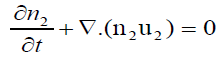(4)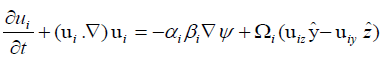(5)(6)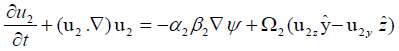(7)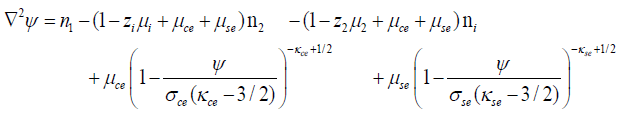(8)

In (2) to (8) ni, n1 and n2 are the hydrogen ion, negative and positive dust number densities, normalized by their equilibrium values ni0, n10 and n20 respectively. ui, u1 and u2 are again, the corresponding fluid speeds all normalized byψ, which is now the dimensionless electric potential, is normalized by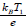. The space and time variables are respectively normalized by the Debye length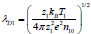and the inverse of the plasma frequency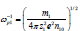. Also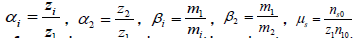and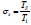, where ns0 is the equilibrium density for species s. Ts and T1 are the temperatures of species s and negative dust respectively. mi, m1 and m2 are, respectively, the masses of hydrogen ions, negatively and positively charged dust particles while Zi (=1), Z1 and Z2 are the corresponding charge numbers.

To investigate nonlinear waves of small amplitude, we derive the K-dV equation with new variables ξ and τ as: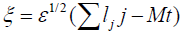where lj denote the direction cosines with j=x, y or z; satisfying the relation, M is the Mach number and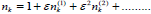.

The physical quantities in the above equations can be expressed asymptotically as a power series in ε about equilibrium values as: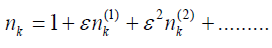(9)(10)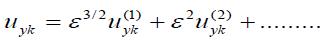(11)(12)(13)

Where k=i (ions), 1 (negatively charged heavier ions/dust) or 2 (positively charged heavier ions/dust) Using ((9)-(13)) in ((2)-(8)) and equating different powers of ε, we can derive the K-dV equation from (8) as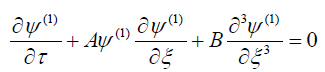(14)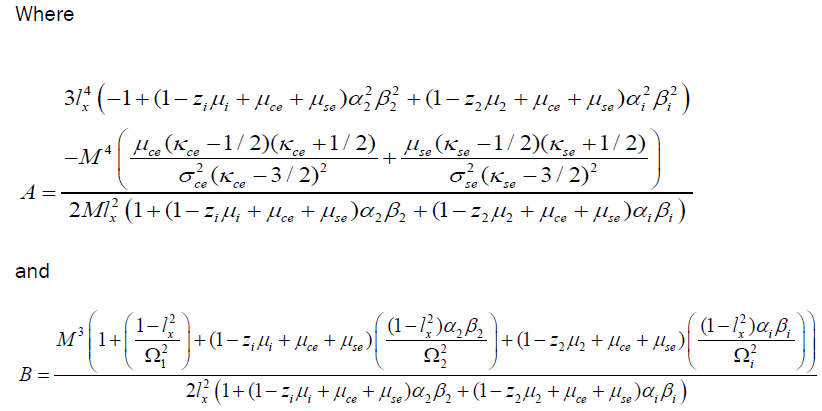Solution of the K-dv Equation

For the solution of the K-dV equation (14), we use the transformationfollowed by Kolebage and Oyewande . The solution of K-dV equation then iswhere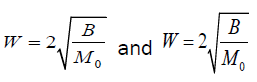are, respectively, the amplitude and width of the soliton.

Results and Discussion

Our equations are valid for arbitrary values of the charge numbers Z1 and Z2 on the heavier/dust particles. However we use, here, parameters observed at comet Halley: the density of hydrogen ions ni0=4.95 cm-3 the temperature of these ions Ti=8 × 104 K and the solar electron temperature Tse=2 × 105 K . The temperature of the secondary photo-electrons was set at Tce=2 × 104 K. The negatively charged heavier ions were detected at energy of the order of 1 eV with densities ≤ 1 cm−3 in the 7-19 amu peaks with negatively charged oxygen ions being unambiguously identified .

Figure 1 is a plot of the solitary wave amplitude ψm versus the direction cosine lx as a function of μi (the normalized hydrogen density). The lower, middle and upper surfaces represent He, C and O ions respectively. The other parameters for the figure are: ly=0.2, Kce=7/2, Kse=13/2, z1=1, z2=2, n10=0.1 cm−3, n20=1 cm−3, Tse=2 × 105 K, Tce=2 × 104 K, THe=1.8 × 104 K, Tc=1.5 × 104 K and TO=1.16 × 104 K. It is clear from the plots that while the amplitude of the soliton increases with increasing obliqueness of propagation; it is almost independent of μi (for higher mass of the heavier particles). Also, the amplitude of the soliton decreases with increase in mass of the heavier species of ions.

Figure 1: Amplitude ψm of the solitary wave vs. direction cosine lx as a function of normalized hydrogen density μi for different heavier pair ions.

Figure 2 is a plot of the solitary wave amplitude ψm versus μse (the normalized solar electron densities) as a function of μi (the normalized hydrogen density). The lower, middle and upper surfaces represent He, C and O ions respectively. The other parameters for the figure are: lx=ly=0.2, Kce=7/2, Kse=13/2, z1=1, z2=2, n10=0.1 cm−3, n20=1 cm−3, Tse=2 × 105 K, Tce=2 × 104 K, THe=1.8 × 104 K, Tc=1.5 × 104 K and TO=1.16 × 104 K. Here also, the amplitude of the soliton decreases with increase in mass of the dust particles. We find that the soliton amplitude is sensitively dependent on the solar electrons, especially for lighter mass of the pair ions.

Figure 2: Amplitude ψm of the solitary wave vs. normalized solar electrons μse as a function of normalized hydrogen density μi for different heavier pair ions.

Next, in Figure 3, we investigate the dependence of the width W of the solitary waves on the normalized solar electron temperatures as a function of the direction cosine lx. The upper, middle and lower surfaces are for He, C and O ions respectively. The other parameters for the figure are: lx=0.2, Kce=7/2, Kse=13/2, z1=1, z2=2, n10=0.1 cm3, n20=1 cm−3, ni0=4.95 cm=3, Tce=2 × 104 K, THe=1.8 × 104 K, Tc=1.5 × 104 K and TO=1.16 × 104 K. The figure shows that the width W is almost independent of σse. However, in all the cases the width is a minimum for parallel and perpendicular directions .

Figure 3: Width W of the solitary wave vs. direction cosine lx as a function of normalized solar electron temperature σse for different heavier pair ions.

Finally, Figure 4 depicts the variation of the width W of the soliton versus kappa indices of solar electrons Kse as a function of kappa indices of commentary electrons Kce for different heavier ions. The lower, middle and upper surfaces is for O, C and He ions respectively. The other parameters for the figure are: lx=ly=0.2, z1=1, z2=2, n10=0.1 cm3, n20=1 cm−3, ni0=4.95 cm−3, Tce=2 × 104 K, THe=1.8 × 104 K, Tc=1.5 × 104 K and TO=1.16 × 104 K. From the plots it is seen that the width of the solitary wave increases as mass of the pair ion increase. Also, the kappa indices of commentary electrons have a stronger influence on the width of the solitary waves as compared to solar electrons.

Figure 4: Width W of the solitary wave vs. kappa indices of solar electrons Kse as a function of kappa indices of commentary electrons Kce for different heavier ions.

Conclusions

We have, in this paper, investigated the influence of ions of different masses on obliquely propagating solitary waves in five component plasma. The five components are hydrogen ions and hot electrons of solar origin; a pair of oppositely charged heavier ions (dust) and colder, photo-electrons of commentary origin. We find that the different pairs of heavier ions strongly influence the amplitude and width of the solitary waves. Our relations can be applicable to a number of space plasma environments [23-27].

Acknowledgments

Financial assistance from the University Grants Commission (EF), Department of Science and Technology (FIST and PURSE Programs) and Kerala State Council for Science, Technology and Environment, Thiruvananthapuram, Kerala (JRFs) is gratefully acknowledged.